# 是不是感觉被淘宝监控了！看啥立马就推送过来！用Python对淘宝用户行为进行分析！

141 篇文章 3 订阅

1.1 数据来源及介绍

## 1.2 数据格式

• User ID: 用户ID
• Item ID: 商品ID
• Category ID: 商品所属品类ID
• Timestamp: 用户行为发生的时间戳

# 二、提出问题

## 2.1 用户行为方面

• 页面浏览量PV和独立访客数UV如何变化？和时间有关系吗？
• 用户的平均访问深度是多少？平台的跳失率怎么样？
• 用户从浏览到购买会经历哪些过程？最终的转化率如何？
• 平台用户留存率怎么样？

## 2.2 用户消费方面

• 平台的用户付费率是多少？用户复购的情况怎么样？
• 平台商品销售情况是怎么样？
• 商品销售之间有没有一定的联系？

## 2.3 用户价值方面

• 如何判断用户价值？针对不同用户如何采取不同的运营策略？

# 三、数据处理

## 3.1 数据导入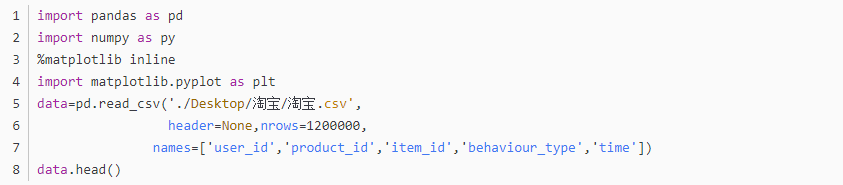​​查看数据类型，是否有缺失值，重复值。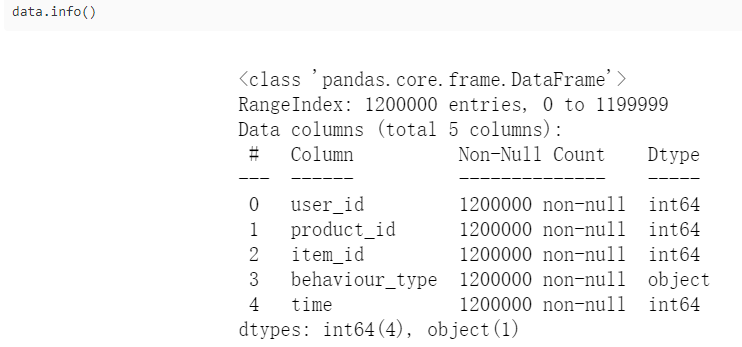​​data.isnull().any()
data.duplicated().sum()

3.2数据整理

import datetime
data['time']=pd.to_datetime(data['time'],unit='s')+datetime.timedelta(hours=8)
data['date']=data['time'].map(lambda x:x.strftime('%Y-%m-%d %H').split(' '))
data['hour']=data['time'].map(lambda x:x.strftime('%Y-%m-%d %H').split(' '))
data['date']=pd.to_datetime(data['date'])
data = data[(data['date'] >= '2017-11-25') & (data['date'] <= '2017-12-03')]
data = data.sort_values(by = ['time','user_id'], ascending=True)
data = data.reset_index(drop=True)
data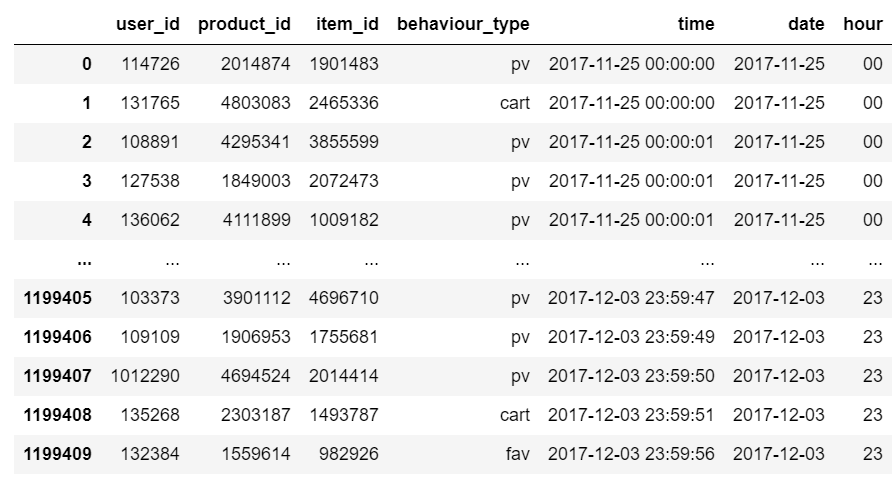​​

# 四、数据分析

（一）用户行为习惯分析

## 4.1.1 页面访问量PV和独立访客数UV

#pv_daily记录每天用户操作次数，uv_daily记录每天不同的上线用户数量
pv_daily=data.groupby('date')['user_id'].count().reset_index().rename(columns={'user_id':'pv'})
uv_daily=data.groupby('date')['user_id'].apply(lambda x:x.drop_duplicates().count()).reset_index().rename(columns={'user_id':'uv'})
x=pv_daily["date"]
y1=pv_daily["pv"]
y2=uv_daily["uv"]
plt.figure(figsize=(10,6))
plt.plot(x,y1,label="pv")
plt.legend(loc=6)
plt.twinx()
plt.plot(x,y2,label="uv",color='r')
plt.legend()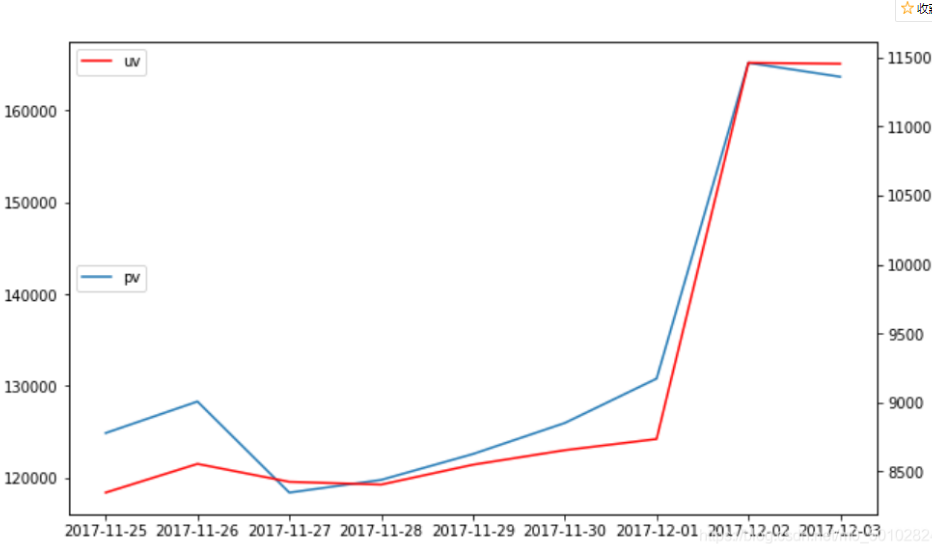​​

接下来按照时间点分析用户的行为习惯：

pv_hour=data.groupby('hour')['user_id'].count().reset_index().rename(columns={'user_id':'pv'})
uv_hour=data.groupby('hour')['user_id'].apply(lambda x:x.drop_duplicates().count()).reset_index().rename(columns={'user_id':'uv'})
x=pv_hour["hour"]
y1=pv_hour["pv"]
y2=uv_hour["uv"]
plt.figure(figsize=(10,6))
plt.plot(x,y1,label="pv")
plt.legend(loc=6)
plt.twinx()
plt.plot(x,y2,label="uv",color='r')
plt.legend(loc=2)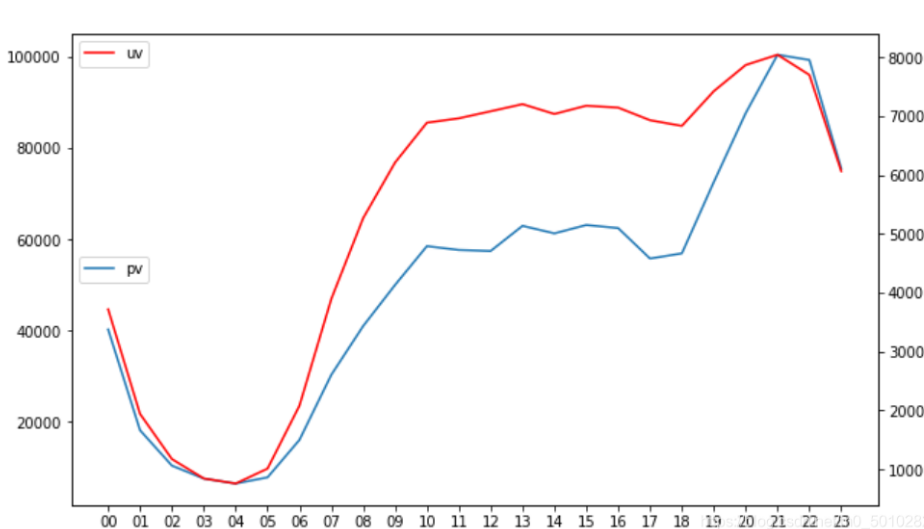​​从上图可发现：从19点开始用户行为数稳步上升，21、22点是一天的最高峰，23点开始有所下滑，1点至7点之间用户行为数都很少，7点后缓慢上升，到中午13点左右有一个小高峰，然后17、18点是个低谷。

## 4.1.2 平均访问深度和跳失率

uv_count = data.user_id.nunique()
pv_count = data[data.behaviour_type == 'pv'].shape
print('平均访问深度是 %.1f' % (pv_count / uv_count))
pv_count_perUser = data[data['behaviour_type'] == 'pv'].groupby('user_id')['behaviour_type'].count().reset_index().rename(columns = {'behaviour_type':'pv_count'})
# 计算只浏览过一次界面的用户数量
bounce_user_count = pv_count_perUser[pv_count_perUser['pv_count'] == 1].shape
print('跳失率是 %.3f%% ' % (100 * (bounce_user_count / uv_count)))

可以得到用户的平均访问深度为92.1，也就是说在11月25到12月3日9天内平均每个用户每天要访问10个界面，可见淘宝用户粘度很高。

按照 跳失率=只浏览一次界面/总用户 计算的话，发现Bounce Rate 只有 0.086% 左右，平台整体流失率相当低。

## 4.1.3 用户转化情况

#不同行为类型用户pv分析
import seaborn as sns
pv_detail=data.groupby(['behaviour_type','hour'])['user_id'].count().reset_index().rename(columns={'user_id':'total_pv'})
plt.subplots(figsize = (10,5))
sns.pointplot(data=pv_detail,hue='behaviour_type',x='hour',y='total_pv')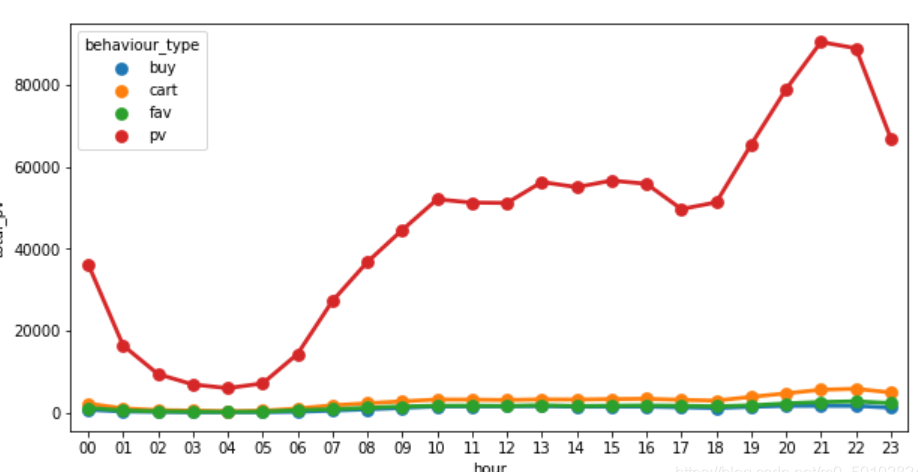​​另外三条不是很清晰，再画一次。

plt.subplots(figsize = (10,5))
sns.pointplot(data=pv_detail[pv_detail.behaviour_type!='pv'],hue='behaviour_type',x='hour',y='total_pv')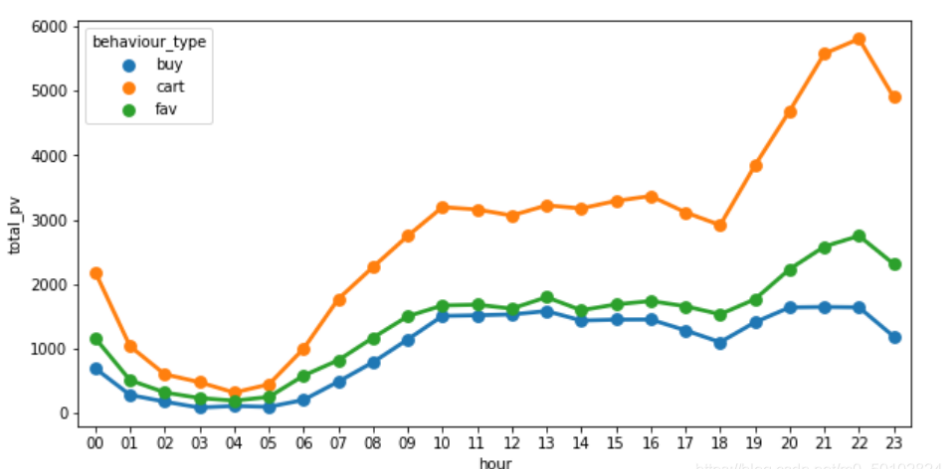​​ 四种用户行为的波动情况基本一致，其中浏览页面pv数远大于其他三项，用户加购物车、收藏和购买数依次降低，可以通过漏斗模型整体分析用户转化情况。

data['behaviour_type'].value_counts().sort_values()
buy       24363
fav       33294
cart      66145
pv      1075608
Name: behaviour_type, dtype: int64

from pyecharts import Funnel
attr_value = [1075608,66145,33294,24363]
fun= Funnel("用户行为漏斗图",title_pos='center')

attr=attrs,                # 指定属性名称
value = attr_value,        # 指定属性所对应的值
is_label_show=True,        # 指定标签是否显示
label_formatter='{c}%',    # 指定标签显示的格式
label_pos="outside",        # 指定标签的位置
legend_orient='vertical',  # 指定图例的方向
legend_pos='left',         # 指定图例的位置
is_legend_show=True)       # 指定图例是否显示

fun.render()
fun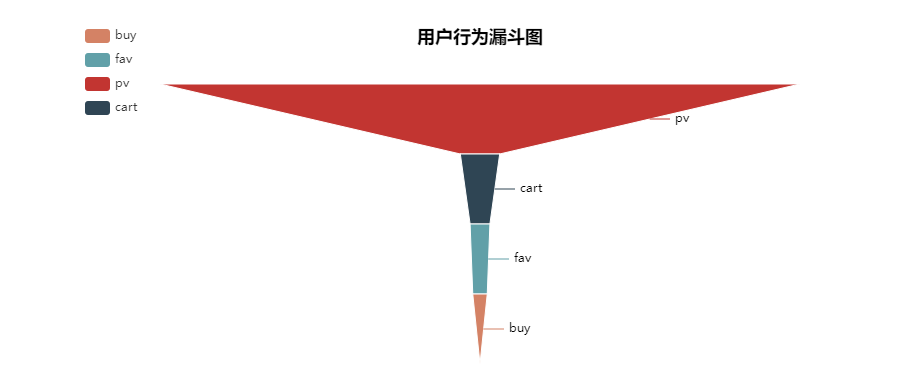​​最后购买的转换率为2.27%左右。

#独立访客数UV
uv=data['user_id'].unique().shape
#有过点击行为的用户数即pv
pv0=data[data["behaviour_type"]=="pv"]["user_id"].drop_duplicates()
pv=pv0.shape
#有过加购物车行为的用户数即cart
cart0=data[data["behaviour_type"]=="cart"]["user_id"].drop_duplicates()
cart=cart0.shape
#有过收藏行为的用户数即fav
fav0=data[data["behaviour_type"]=="fav"]["user_id"].drop_duplicates()
fav=fav0.shape
#每个行为的独立用户数
uniqueuser
uv      11675
pv      11636
cart     8775
fav      4603
dtype: int64

attrs = ['uv','pv','cart','fav','buy']
attr_value = uniqueuser.values
fun1= Funnel("用户行为漏斗图",title_pos='center')

attr=attrs,                # 指定属性名称
value = attr_value,        # 指定属性所对应的值
is_label_show=True,        # 指定标签是否显示
label_formatter='{c}%',    # 指定标签显示的格式
label_pos="outside",        # 指定标签的位置
legend_orient='vertical',  # 指定图例的方向
legend_pos='left',         # 指定图例的位置
is_legend_show=True)       # 指定图例是否显示

fun1.render()
fun1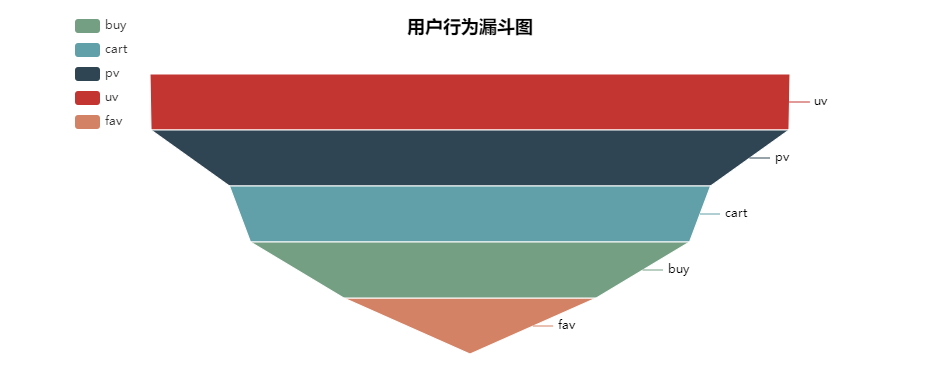​​可以看到uv到最终购买的转化率为68.58%，流失率为31.42%。

上面近32%的“流失用户”中，有的可能在不久的将来还会成为购买用户，因为有的加购或收藏了商品；但是上文提到的“跳失用户”只进行了一次点击，再成为活跃用户的可能性很小。

（二）用户消费习惯分析

## 4.2.1 用户付费率PUR（Paying User Rate）

# 用户付费率分析

print('用户付费率是 %.2f%%' % ( 100 * paying_user_count / uv_count))
用户付费率是 68.58%

## 4.2.2 用户购买次数

# 用户购买次数情况分析
import matplotlib.pyplot as plt
#用户，购买次数
#根据次数再分组画图
plt.bar(ub.index,ub.values)


cnt
1     2699
2     1876
3     1253
4      742
5      460
6      299
7      209
8      123
9       85
10      58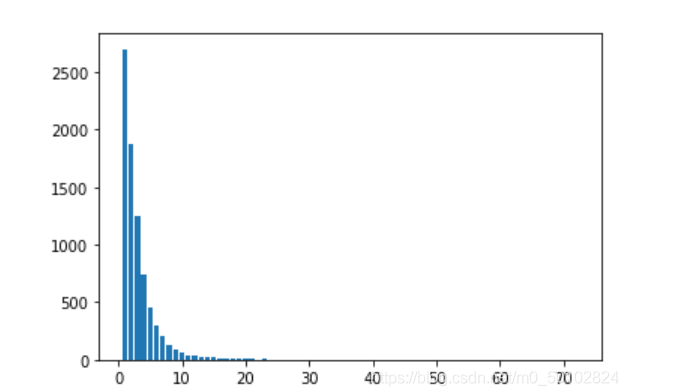​​由上图可知，购买次数为1次的用户数量最多

## 4.2.3 用户复购率

#复购用户数量 通过筛选支付次数>=2
print('复购率是 %.2f%%' % (100 * paying_retention_user_count / paying_user_count))
复购率是 66.29%

## 4.2.4 用户复购的间隔时间

#计算用户复购的间隔时间


user_id
100      1        2
2        1
117      4        1
5        1
6        1
..
1017938  15947    1
1017960  15950    4
15951    2
1017972  15954    4
1017997  15956    6
Name: date, Length: 7951, dtype: int64


buy_day.value_counts().plot(kind='bar')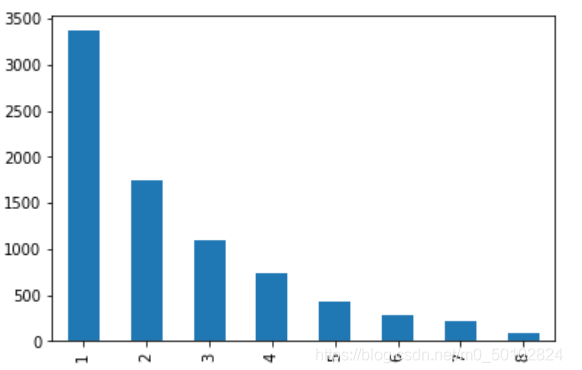​​

## 4.2.5 商品销售分析

（1）先看“item_id”的点击量和购买量整体情况：

pv_item=data[data["behaviour_type"]=="pv"].groupby("item_id")["user_id"].count().sort_values(ascending=False)
x=merge["user_id_x"]
y=merge["user_id_y"]
plt.figure(figsize=(8,6))
plt.scatter(x,y,marker="o")
#商品类目点击量和购买量关系图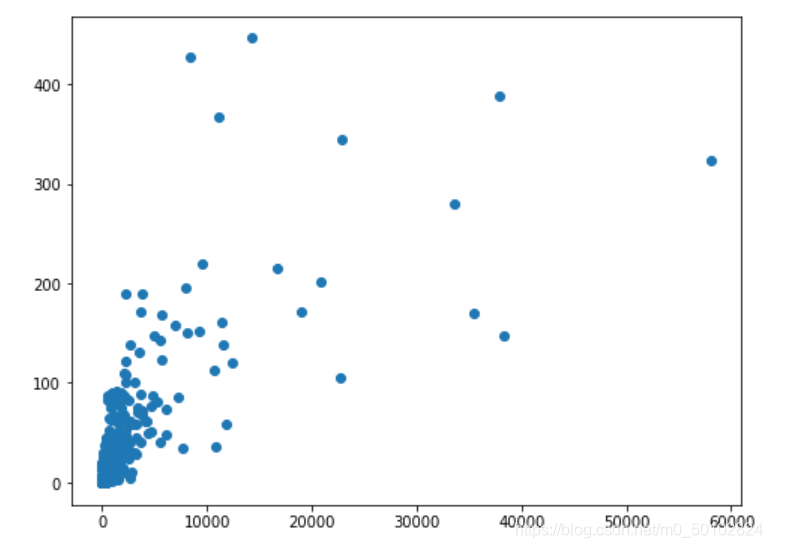​​从点击量和购买量的散点图可以看出，大部分商品类目都是点击量和购买量都比较低，整体是正相关，有少量类目的点击量或购买量很大，或点击量和购买量都比较大。

（2）再看“product_id”的点击量和购买量整体情况：

pv_product=data[data["behaviour_type"]=="pv"].groupby("product_id")["user_id"].count().sort_values(ascending=False)
x=merge["user_id_x"]
y=merge["user_id_y"]
plt.figure(figsize=(8,6))
plt.scatter(x,y,marker="o")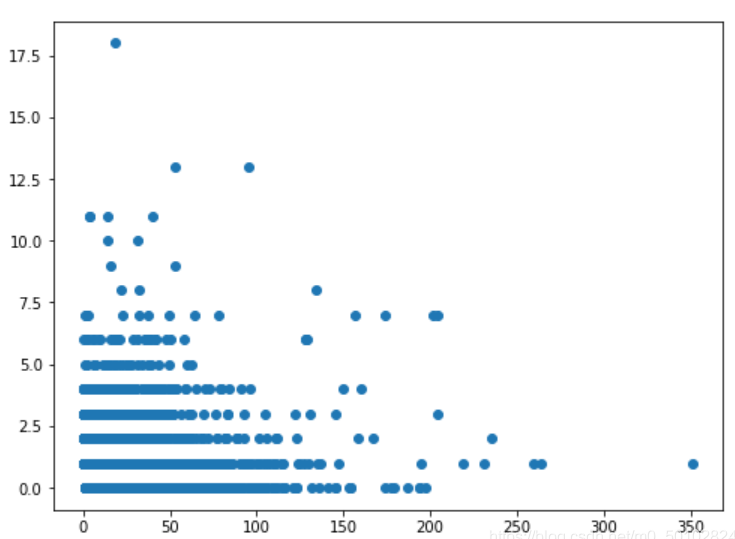​​同商品类目的情况类似，大部分商品是点击量和购买量都比较低，有一定的相关性，但同时发现点击量大但购买量小的商品较多，即偏右下、偏右中的点，有一个左上角突出的点，表示有一个商品点击量不大但购买量很大。

（3)下面分析商品类目 点击量和购买量的前十：

buytop=pd.pivot_table(data,values="user_id",columns="behaviour_type",index="item_id",aggfunc="count").sort_values(by=["buy"],ascending=False).head(10)
buytop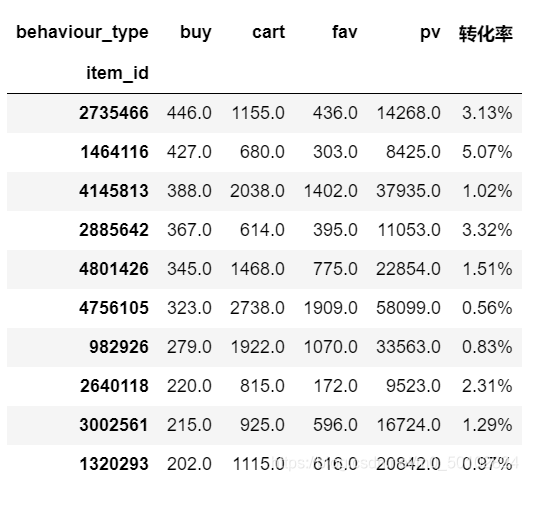​​

pvtop=pd.pivot_table(data,values="user_id",columns="behaviour_type",index="item_id",aggfunc="count").sort_values(by=["pv"],ascending=False).head(10)
pvtop['转化率']=pvtop['转化率'].apply(lambda x: format(x, '.2%'))
pvtop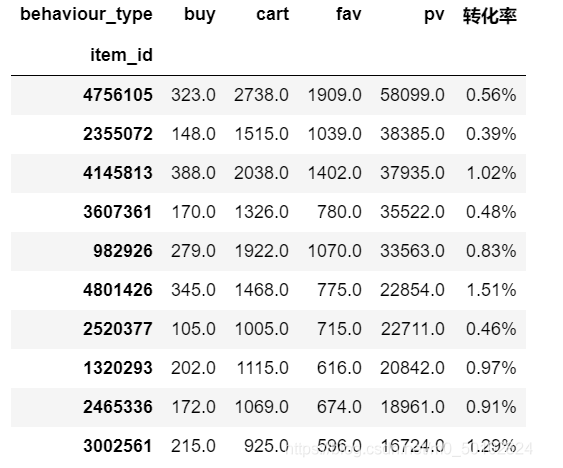​​

pd.merge(buytop,pvtop,how='inner',on='item_id')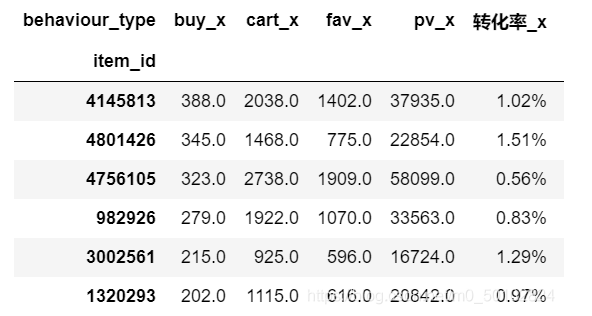​​

这6个类目的点击量和购买量都是前十，说明是很受用户欢迎的类目，无疑是重点类目，但发现其实这些类目的转化率也不是很高，电商平台应该重点推送这几类商品，因为这类商品有市场，并且可以多做活动，吸引更多的潜在客户变成购买客户。

而购买量比较高，但是点击量不突出的这类商品，也许是特定群体非常需要的，他们可能搜索和点击的目标比较明确。电商平台可以收集用户信息，分析用户画像，结合商品特点，核实是否如此。

但是点击量很高，但购买量偏低的这类商品，应该先从商品本身分析，是否是用户真正需要的。为什么大部分用户点击，但购买较少，是因为价格太高？还是其他原因？调查清楚原因之后再对症下药。

RFM模型的概念如下： R（Recency）：客户最近一次交易时间的间隔。R值越大，表示客户交易发生的日期越久，反之则表示客户交易发生的日期越近。

F（Frequency）：客户在最近一段时间内交易的次数。

F值越大，表示客户交易越频繁，反之则表示客户交易不够活跃。

M（Monetary）：客户在最近一段时间内交易的金额。

M值越大，表示客户价值越高，反之则表示客户价值越低。

由于所给数据集不包含用户购买金额，所以只考虑最近消费时间R和购买频率F，将客户分为重要客户、保持客户、发展客户和挽留客户。

# 1 R: 最近一次消费距今天数统计
nowDate = datetime.datetime(2017,12,4) # 假定当前时间为（2017，12，4）
user_recent_pay = data[data['behaviour_type'] == 'buy'].groupby('user_id')['date'].apply(lambda x: nowDate - x.sort_values().iloc[-1])
user_recent_pay = user_recent_pay.reset_index().rename(columns={'date':'recent'})

# 2 F: 消费次数统计
user_freq = user_freq.reset_index().rename(columns={'date':'freq'})

# 3 通过user_id将R、F合并
rfm = pd.merge(user_recent_pay, user_freq, left_on='user_id', right_on='user_id')

# 4 给R、F打分score
rfm['score_recent'] = pd.qcut(rfm['recent'], 2, labels = ['1', '0'])
rfm['score_freq'] = pd.qcut(rfm['freq'], 2, labels = ['0', '1'])

# 5 得分拼接
rfm['rfm'] = rfm['score_recent'].str.cat(rfm['score_freq'])

# 6 根据RFM分类
rfm = rfm.assign(user_type = rfm['rfm']
.map({'11':'重要客户', '01':'保持客户', '10':'发展客户', '00':'挽留客户'}))

pi=rfm.groupby('user_type')['user_id'].count()
pi

user_type

plt.figure(1, figsize=(6,6))
plt.pie([951,2131,2444,2481],labels=['保持客户','发展客户','挽留客户','重要客户'],autopct='%1.2f%%')
plt.title("R F M")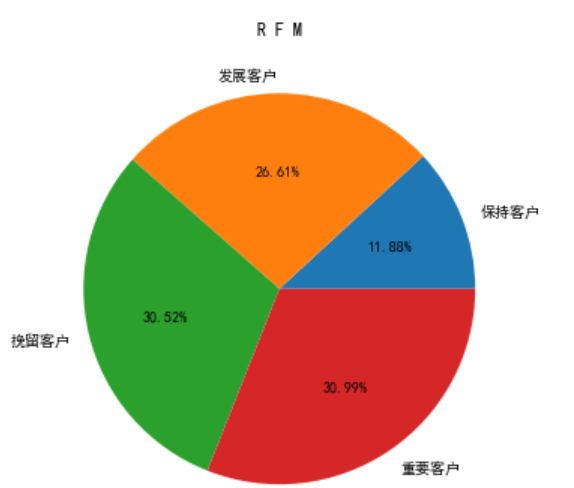​​

## 结束

05-152万+11-082502
03-067136
01-12757
06-052万+
09-25542
07-02363
06-07186
08-05118
10-2915
10-152622
03-265313
11-10318
04-21763
04-17914
11-15672
12-21871
11-03763
11-051062点击重新获取扫码支付1.余额是钱包充值的虚拟货币，按照1:1的比例进行支付金额的抵扣。
2.余额无法直接购买下载，可以购买VIP、C币套餐、付费专栏及课程。余额充值